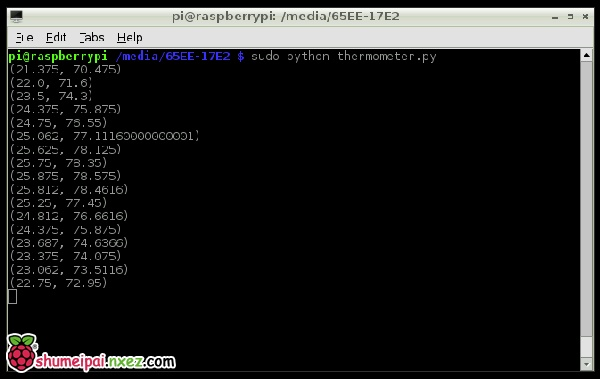# 树莓派教程：DS18B20温度传感器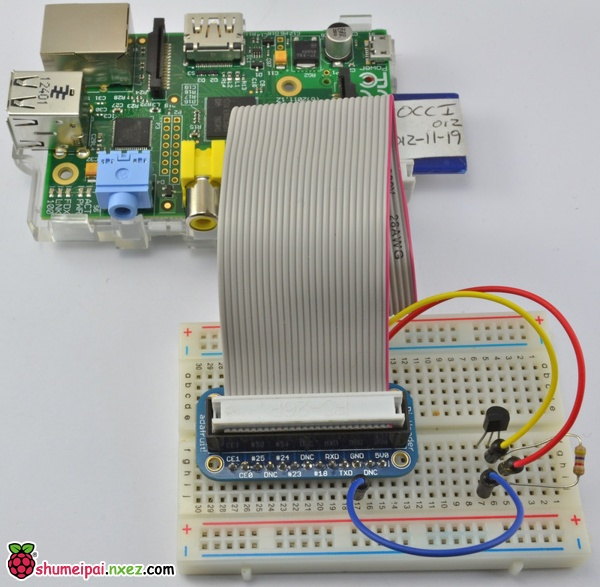#### 你需要的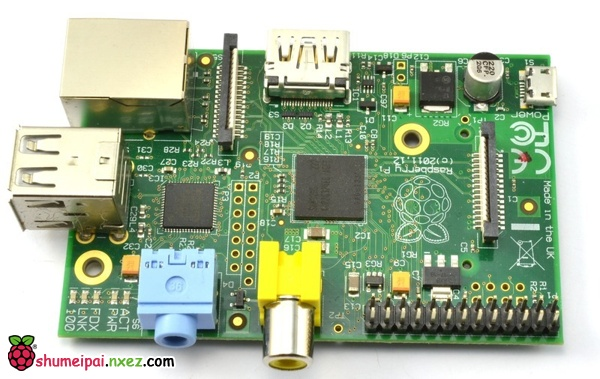DS18B20数字温度传感器 + 延长线，也可以是湿度或者高温型号的。另外你需要一个4.7k或者10k欧姆的电阻。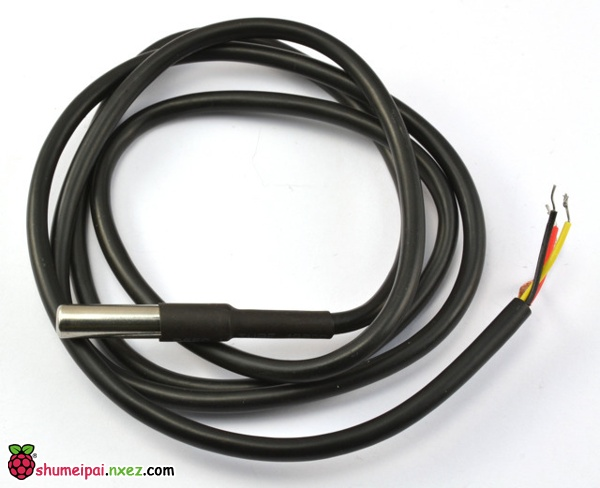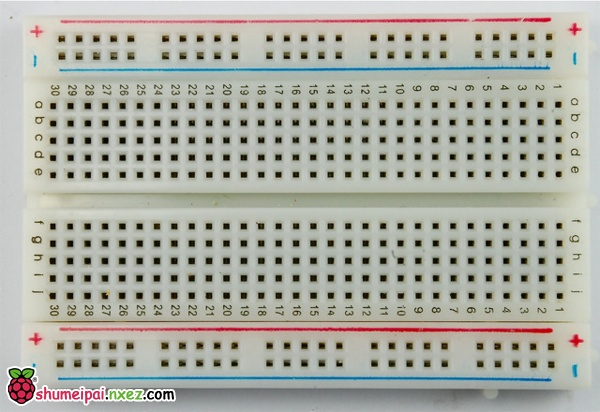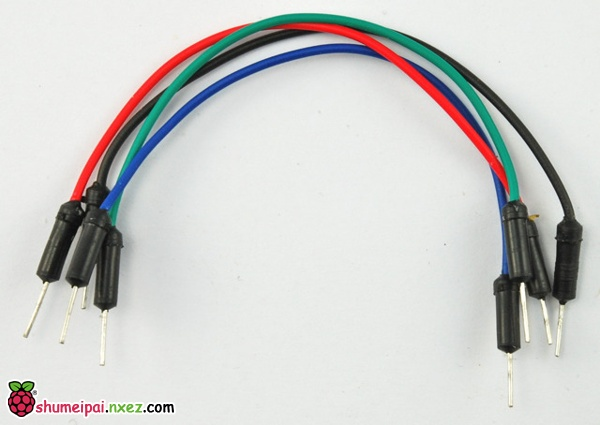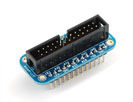#### 硬件部分

DS18B20单总线(1-wire)传感器可以以非并行连接接入，这一点几乎与其他市售的传感器都不同！所有传感器共享相同的针脚，所以你只需要一个4.7k的电阻就够了。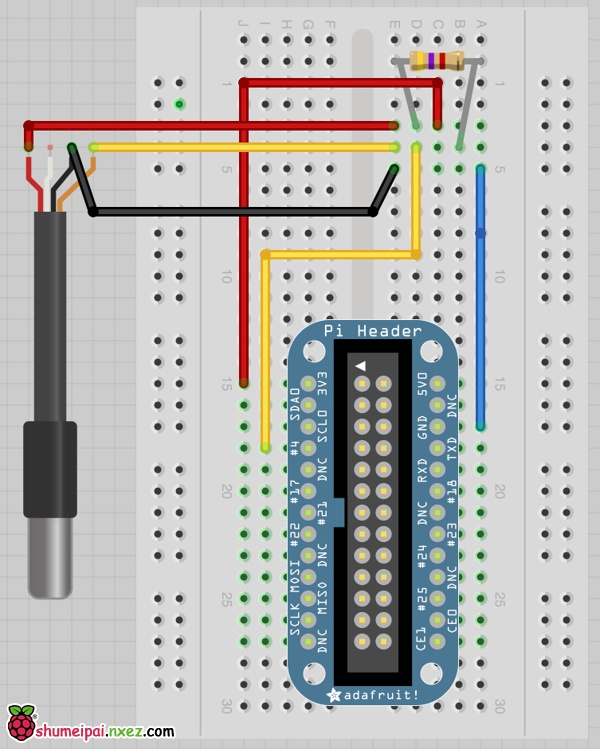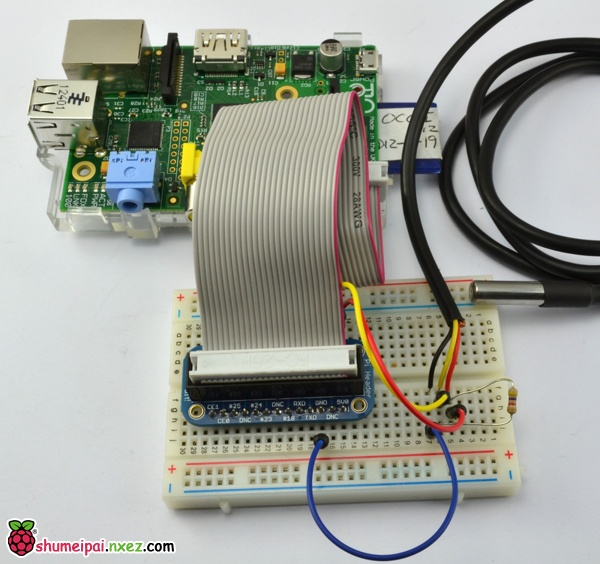#### DS18B20

 1 2 3 4 5 6 `sudo` `modprobe w1-gpio` `sudo` `modprobe w1-therm` `cd` `/sys/bus/w1/devices` `ls` `cd` `28-xxxx (change this to match what serial number pops up)` `cat` `w1_slave`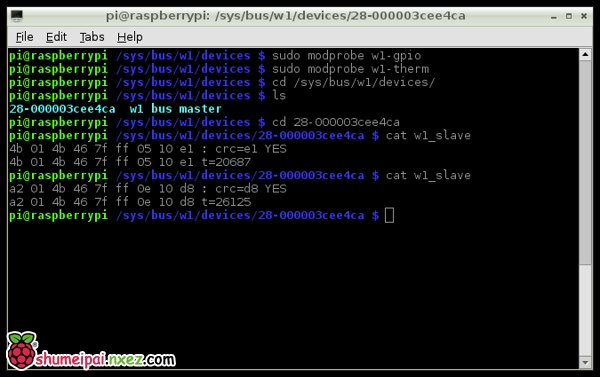#### 软件部分

 1 2 3 4 5 6 7 8 9 10 11 12 13 14 15 16 17 18 19 20 21 22 23 24 25 26 27 28 29 30 31 32 `import` `os` `import` `glob` `import` `time` ` ` `os.system(``'modprobe w1-gpio'``)` `os.system(``'modprobe w1-therm'``)` ` ` `base_dir ``=` `'/sys/bus/w1/devices/'` `device_folder ``=` `glob.glob(base_dir ``+` `'28*'``)[``0``]` `device_file ``=` `device_folder ``+` `'/w1_slave'` ` ` `def` `read_temp_raw():` `    ``f ``=` `open``(device_file, ``'r'``)` `    ``lines ``=` `f.readlines()` `    ``f.close()` `    ``return` `lines` ` ` `def` `read_temp():` `    ``lines ``=` `read_temp_raw()` `    ``while` `lines[``0``].strip()[``-``3``:] !``=` `'YES'``:` `        ``time.sleep(``0.2``)` `        ``lines ``=` `read_temp_raw()` `    ``equals_pos ``=` `lines[``1``].find(``'t='``)` `    ``if` `equals_pos !``=` `-``1``:` `        ``temp_string ``=` `lines[``1``][equals_pos``+``2``:]` `        ``temp_c ``=` `float``(temp_string) ``/` `1000.0` `        ``temp_f ``=` `temp_c ``*` `9.0` `/` `5.0` `+` `32.0` `        ``return` `temp_c, temp_f` ` ` `while` `True``:` `    ``print``(read_temp())   ` `    ``time.sleep(``1``)`

 1 2 3 4 5 6 `def` `read_temp_raw():` `    ``catdata ``=` `subprocess.Popen([``'cat'``,device_file], stdout``=``subprocess.PIPE, stderr``=``subprocess.PIPE)` `    ``out,err ``=` `catdata.communicate()` `    ``out_decode ``=` `out.decode(``'utf-8'``)` `    ``lines ``=` `out_decode.split(``'\n'``)` `    ``return` `lines`

 1 `deg_c, deg_f ``=` `read_temp()`

 1 `nano thermometer.py`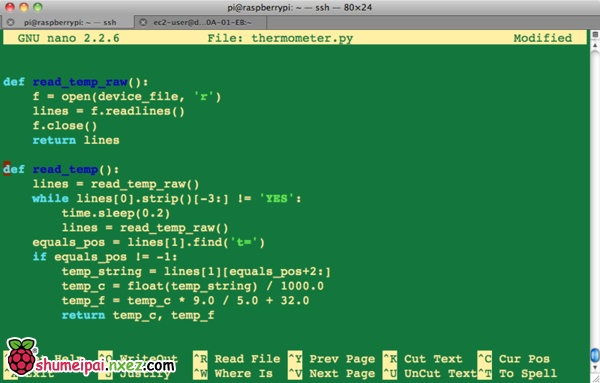#### 测试与配置

 1 `sudo` `python thermometer.py`